# Electronics and Communication Engineering - Exam Questions Papers

41.

The system shown in figure remains stable when :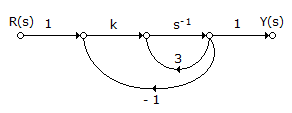A. k £ - 1 B. -1 < k < 1 C. l < k < 3 D. k > 3

Explanation:

Pi = 1 x k x s-1 x 1 ⇒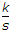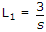, L2 = k x s-1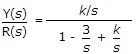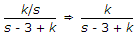System will be stable when k - 3 > 0 or k > 3.

42.

The mechanical system shown below has its pole(s) at: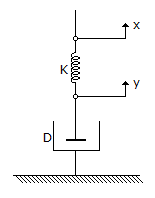A. - K/D B. - D/K C. - DK D. 0, - K/D

Explanation:Kx(s) - Ky(s) = Dsy(s)

Kx(s) = Dsy(s) + Ky(s)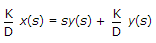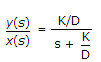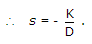43.

The step error coefficient of a system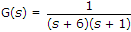with unity feedback is :

 A. 1/6 B. ∞ C. 0 D. 1

Explanation:

kp = s → 0 G(s)H(s).

44.

The following k-map implements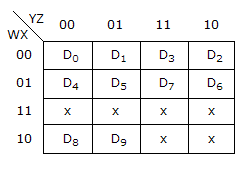A. BCD to Decimal Decoder B. Decimal to BCD Encoder C. 1 of 10 Decoder D. (a) and (c)

Explanation:

As the K-map reveals W, X, Y, Z are the input lines to the device and D0 to D9 are the output lines which get activated depending upon the input combinations.

As seen from k-map for W, X, Y, Z from 0000 to 1001 we have D0 to D9 outputs activated respectively.

This operation is performed by BCD to Decimal decoder.

Also it is called as 1 off 10 decoder, as only 1 of 10 output line is high.

45.

The Z-transform of a signal is given by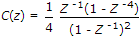its final value is __________ .

 A. infinite B. 1/4 C. zero D. 1

Explanation: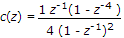Final value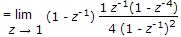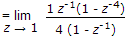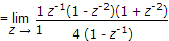Final value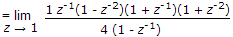Final value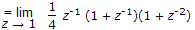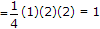.

#### Current Affairs 2022

Interview Questions and Answers